# Lewis Structures (to be fixed)

$$\newcommand{\vecs}{\overset { \rightharpoonup} {\mathbf{#1}} }$$ $$\newcommand{\vecd}{\overset{-\!-\!\rightharpoonup}{\vphantom{a}\smash {#1}}}$$$$\newcommand{\id}{\mathrm{id}}$$ $$\newcommand{\Span}{\mathrm{span}}$$ $$\newcommand{\kernel}{\mathrm{null}\,}$$ $$\newcommand{\range}{\mathrm{range}\,}$$ $$\newcommand{\RealPart}{\mathrm{Re}}$$ $$\newcommand{\ImaginaryPart}{\mathrm{Im}}$$ $$\newcommand{\Argument}{\mathrm{Arg}}$$ $$\newcommand{\norm}{\| #1 \|}$$ $$\newcommand{\inner}{\langle #1, #2 \rangle}$$ $$\newcommand{\Span}{\mathrm{span}}$$ $$\newcommand{\id}{\mathrm{id}}$$ $$\newcommand{\Span}{\mathrm{span}}$$ $$\newcommand{\kernel}{\mathrm{null}\,}$$ $$\newcommand{\range}{\mathrm{range}\,}$$ $$\newcommand{\RealPart}{\mathrm{Re}}$$ $$\newcommand{\ImaginaryPart}{\mathrm{Im}}$$ $$\newcommand{\Argument}{\mathrm{Arg}}$$ $$\newcommand{\norm}{\| #1 \|}$$ $$\newcommand{\inner}{\langle #1, #2 \rangle}$$ $$\newcommand{\Span}{\mathrm{span}}$$$$\newcommand{\AA}{\unicode[.8,0]{x212B}}$$

Lewis Structures are visual representations of the bonds between atoms and illustrate the lone pairs of electrons in molecules. They can also be called Lewis dot diagrams and are used as a simple way to show the configuration of atoms within a molecule. Between 1916 and 1919, Gilbert Newton Lewis, Walther Kossel, and Irving Langmuir came up with a theory to explain chemical bonding. This theory would be later called Lewis Theory and it is based on the following principles:

1. Valence electrons, or the electrons in the outermost electron shell, have an essential role in chemical bonding.
2. Ionic bonds are formed between atoms when electrons are transferred from one atom to another. Ionic bond is a bond between nonmetals and metals .
3. Covalent bonds are formed between atoms when pairs of electrons are shared between atoms. A covalent bond is between two nonmetals.
4. Electrons are transferred/shared so that each atom may reach a more stable electron configuration i.e. the noble gas configuration which contains 8 valence electrons. This is called octet rule.

## Lewis Symbols and Lewis Structures

A Lewis Symbol for an element is composed of a chemical symbol surrounded by dots that are used to represent valence electrons. An example of a Lewis symbol is shown below with the element Carbon, which has the electron configuration of 1s22s22p2: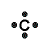This Lewis symbol shows that carbon has four valence electrons in its outer orbital and these four electrons play a major role in bonding of carbon molecules.

Lewis symbols differ slightly for ions. When forming a Lewis symbol for an ion, the chemical symbol is surrounded by dots that are used to represent valence electrons, and the whole structure is placed in square brackets with superscript representing the charge of the ion. An example of a Lewis symbol for the cation and anion of Carbon is shown below:

Cation of Carbon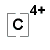Anion of Carbon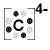## Constructing Lewis Structures

To construct Lewis Structures one can generally abide by the following steps:

1. Find how many valence electrons (N) are in the molecule that needs to be shown on the Lewis Structure by using the periodic table. Find the charge, add an electron for every negative charge and remove an electron for every positive charge.
2. Draw out the single bonds and initial framework, called the skeleton, of the molecule.
3. Complete the octets around the non-central atoms i.e. the terminal atoms by using the lone-pairs of electrons.
4. Compare the number of electrons currently depicted to the number needed (N) in the central atom and add electrons to it if less the number is less than N.
5. If there are extra lone-pair electrons and the octet rule is not filled for the central atom, use the extra electrons to form double or triple bonds around the central atom.
6. Check the formal charge of each atom (Formal Charge explained below).

When constructing the structures keep in mind the following:

• The dots surrounding the chemical symbol are the valence electrons, and each dash represents one covalent bond (consisting of two valence electrons)
• Hydrogen is always terminal in the structure
• The atom with the lowest ionization energy is typically the central atom in the structure
• The octet rule means there are 8 valence electrons around the atoms, but for hydrogen the maximum is 2 electrons

Lewis Structures can differ based on whether the electrons are shared through ionic or covalent bonds.

Example 1: Ionic Bonding in NaCl

An example of ionic bonding can be seen below in the instance of the reaction of Sodium and Chlorine:

$2Na_{(s)} + 2Cl_{2 (g)} \rightarrow 2NaCl_{s}$

Sodium has one valence electron and Chlorine has seven valence electrons; the two elements together form the noble gas configuration. The Chlorine atom takes the valence electron from the Sodium atom leaving the Chlorine atom with one extra electron and thus negatively charged and the Sodium atom without an electron and thus positively charged. The two atoms then become ions and because of their opposite charges the ions are held together in an ionic bond.

An example of covalent bonding can be seen below with the reaction of Hydrogen and Fluorine: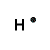+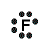=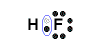Hydrogen has one valence electron and Fluorine has seven valence electrons; together the elements form the noble gas configuration. The Hydrogen atom shares its electron with Fluorine atom so that the Hydrogen atom has 2 electrons and the Fluorine atom has 8 electrons. Therefore both atoms have their outermost shells completely filled.

Example 2: The Chlorate Ion

Try the Chlorate ion: (ClO3-)

SOLUTION

First, lets find the how many valence electrons chlorate has:

ClO3- : 7 e-(from Cl) + 3(6) e-(from 3 O atoms) + 1 (from the total charge of -1) = 26

There are 26 valence electrons.

Next lets draw the basic framework of the molecule: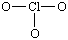The molecule uses covalent bonds to hold together the atoms to the central Chlorine. The remaining electrons become non-bonding electrons. Since 6 electrons were used for the bonds, the 20 others become those un-bonding electrons to complete the octet:

The oxygen atom's shells fill up with 18 electrons, and the other 2 complete Chlorine's octet.Example 3: Formaldehyde

Constructing the Lewis Structure of the formaldehyde (H2CO) molecule.

SOLUTION

First find the valence electrons:

H2CO: 2(1) e- (from the H atoms) + 4 e- (from the C atom) + 6 e- (from the O atom)

There are 12 valence electrons. Next draw out the framework of the molecule:To satisfy the octet of Carbon, one of the pairs of electrons on Oxygen must be moved to create a double bond with Carbon. Therefore our Lewis Structure would look as it does below: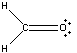The Hydrogen atoms are each filled up with their two electrons and both the Carbon and the Oxygen atoms' octets are filled.

## Formal Charge

The charge on each atom in a molecule is called the formal charge. The formal charge can be calculated if the electrons in the bonds of the molecule are equally shared between atoms. This is not the same thing as the net charge of the ion.

In calculating formal charge, the following steps can be extremely helpful:

1. Determine the number of valence electrons that should be present for each atom in the structure.
2. Count the electrons around each atom in the structure (each lone pair = 2 electrons, each single bond =1 electron, each double bond = 2 electrons, each triple bond = 3 electrons).
3. Subtract the number of valence electrons that should be present (from step 1) from the electrons counted in step 2 for each atom. This is the formal charge for each atom.
4. Check that the formal charges add up to equal the overall charge of the molecule.

Formal charge = (number of valence electrons) - (number of non-bonding electrons + 1/2 number of bonding electrons)

In Lewis structures, the most favorable structure has the smallest formal charge for the atoms, and negative formal charges tend to come from more electronegative atoms.

An example of determining formal charge can be seen below with the nitrate ion, NO3-:• The double bonded O atom has 6 electrons: 4 non-bonding and 2 bonding (one electron for each bond). Since O should have 6 electrons, the formal charge is 0.
• The two singly bonded O atoms each have 7 electrons: 6 non-bonding and 1 bonding electron. Since O should have 6 electrons, and there is one extra electron, those O atoms each have formal charges of -1.
• The N atom has 4 electrons: 4 bonding and 0 non-bonding electrons. Since N should have 5 electrons and there are only 4 electrons for this N, the N atom has a formal charge of +1.
• The charges add up to the overall charge of the ion. 0 + (-1) + (-1) + 1 = -1. Thus, these charges are correct, as the overall charge of nitrate is -1.

## Resonance

There are times when more than one acceptable Lewis structure can be drawn for a molecule and no single structure can represent the molecule entirely. When this occurs the molecule/ion is said to have resonance. The combination of the various plausible Lewis structures is called a resonance hybrid.

Some rules for drawing resonance structures are as follows:

1. The same number of electrons must be present for all resonance structures.
2. The octet rule must be obeyed.
3. Nuclei (or the chemical symbol in the structure's representation) cannot be rearranged; only the valence electrons differ for resonance structures.

Example 4: Nitrate Ion

Consider the nitrate ion, NO3-• Each structure differs based on the movement of two electrons.
• From structure 1 to structure 2 one of the lone pairs on the blue O moves to form a double bond with N. One of the electrons shared in the double bond between the red O and N then moves to be a lone pair on the red O because the N cannot have 10 electrons surrounding it.
• This is the same process that occurs from structure 2 to structure 3 except the changes occur in the green O and blue O.

## Problems

1. Draw the Lewis structure for water.
2. Find the formal charge for each of the atoms in water.
3. Draw the Lewis structure for ammonia.
4. Find the formal charge for each of the atoms in ammonia.

1.2. All atoms in water have a formal charge of 0.

3.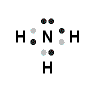4. The formal charge for each H is 0 and for N is 0.

## References

1. Zumdahl, Steven S., et al. Chemistry. Boston, MA: Houghton Mifflin, 1997.
2. Petrucci, Ralph H., et al. General Chemistry: Principles and Modern Applications. Upper Saddle River, NJ: Pearson Prentice Hall, 2007.